Question

# What accounts for the lower boiling point of the compound that distills first? a) It has...

What accounts for the lower boiling point of the compound that distills first?
a) It has the least atoms
b) It exhibits less hydrogen bonding
c) It is lighter
d) It is the smallest molecule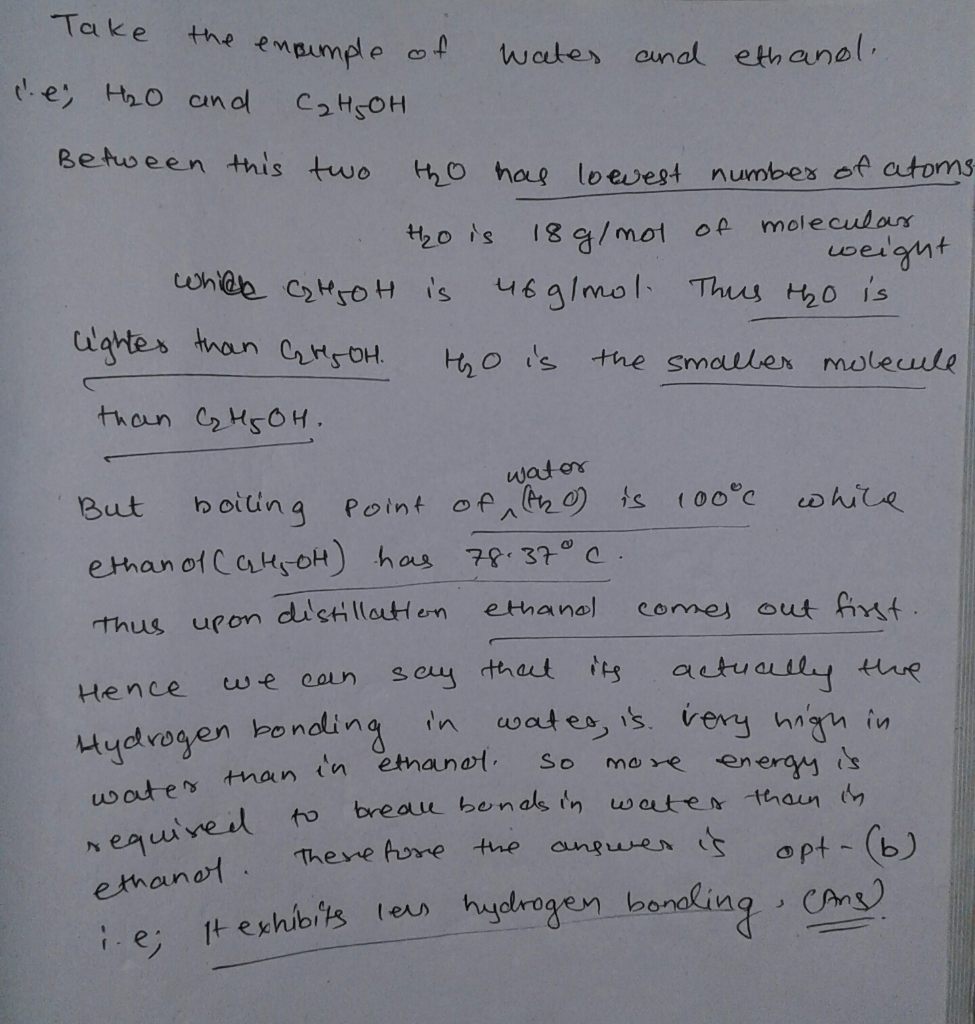#### Earn Coins

Coins can be redeemed for fabulous gifts.

Similar Homework Help Questions
• ### These two molecules have an identical number of electrons. However, the boiling point of Compound B...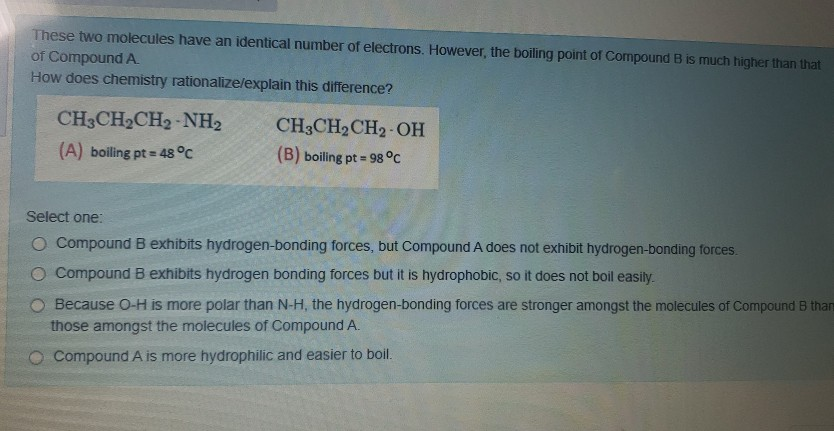These two molecules have an identical number of electrons. However, the boiling point of Compound B is much higher than that of Compound A How does chemistry rationalizelexplain this difference? CH3CH2CH2-NH2 (A) boiling pt = : 48 °C CH3CH2CH2-OH (B) boiling pt = 98°C Select one: O Compound B exhibits hydrogen-bonding forces, but Compound A does not exhibit hydrogen-bonding forces O Compound B exhibits hydrogen bonding forces but it is hydrophobic, so it does not boil easily. Because O-H is...

• ### Comparing the boiling points of: A) C3H8 B) C5H12 C) C2H5OH and identify the folowing statements as either True or False: Dispersion forces only for (A) Boiling point (C) > Boiling point (A) (B) exhibits hydrogen bonding Boiling p

Comparing the boiling points of: A) C3H8 B) C5H12 C) C2H5OH and identify the folowing statements as either True or False: Dispersion forces only for (A) Boiling point (C) > Boiling point (A) (B) exhibits hydrogen bonding Boiling point (C) < Boiling point (A) (A) exhibits hydrogen bonding Boiling point (A) < Boiling point (B) Boiling point (B) < Boiling point (C) Dispersion forces only for (C) Boiling point (B) > Boiling point (C) Dispersion forces only for (B) (C)...

• ### Part A H,0 (100°C) has a higher boiling point than CH, OH (65°C). There are more...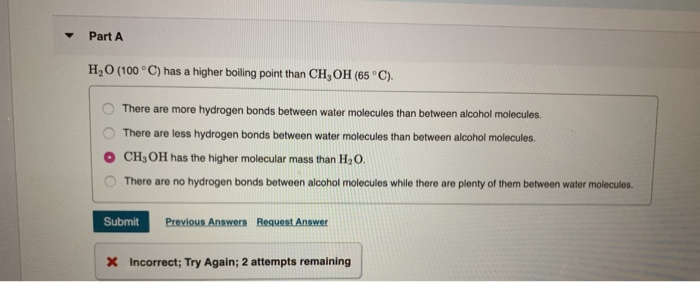Part A H,0 (100°C) has a higher boiling point than CH, OH (65°C). There are more hydrogen bonds between water molecules than between alcohol molecules. There are less hydrogen bonds between water molecules than between alcohol molecules. CH, OH has the higher molecular mass than H,O. There are no hydrogen bonds between alcohol molecules while there are plenty of them between water molecules. O Submit Previous Answers Request Answer X Incorrect; Try Again; 2 attempts remaining Part B H,O (100°C)...

• ### Question 6 3 pts Select whether compound A or compound B will have the higher boiling...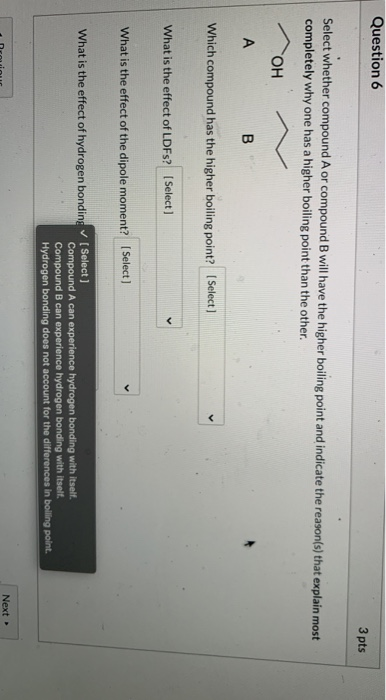Question 6 3 pts Select whether compound A or compound B will have the higher boiling point and indicate the reason(s) that explain most completely why one has a higher boiling point than the other. OH А B Which compound has the higher boiling point? (Select] What is the effect of LDFS? (Select) What is the effect of the dipole moment? (Select) What is the effect of hydrogen bonding (Select] Compound A can experience hydrogen bonding with itself. Compound B...

• ### C The boiling point of H,O is much higher than the boiling point of HS. H2O...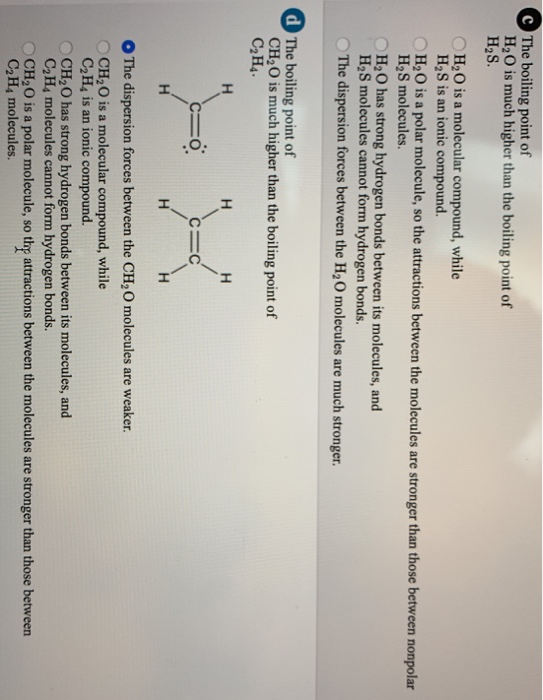C The boiling point of H,O is much higher than the boiling point of HS. H2O is a molecular compound, while HS is an ionic compound. H2O is a polar molecule, so the attractions between the molecules are stronger than those between nonpolar H2S molecules. H2O has strong hydrogen bonds between its molecules, and HS molecules cannot form hydrogen bonds. The dispersion forces between the H2O molecules are much stronger. d The boiling point of CH,O is much higher than...

• ### Ethane (molar mass 30.07 g/mol) boils at-88.6℃ Methanol has almost the same molar mass (32.04 g...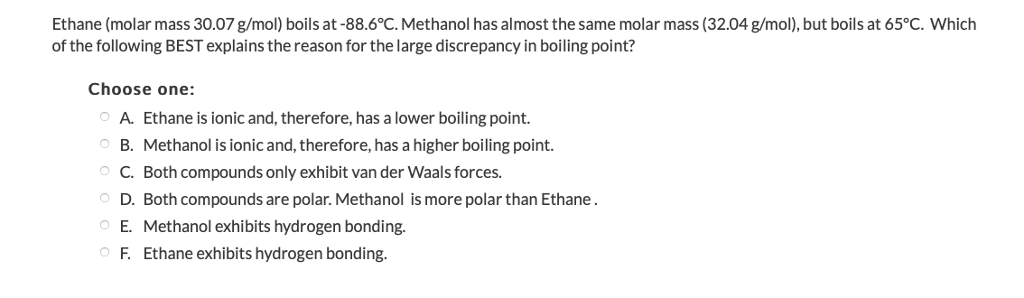Ethane (molar mass 30.07 g/mol) boils at-88.6℃ Methanol has almost the same molar mass (32.04 g mol), but boils at 65℃, which of the following BEST explains the reason for the large discrepancy in boiling point? Choose one: A. Ethane is ionic and, therefore, has a lower boiling point. B. Methanol is ionic and, therefore, has a higher boiling point. C. Both compounds only exhibit van der Waals forces. D. Both compounds are polar. Methanol is more polar than Ethane....

• ### Arrange the compounds in order of increasing boiling point

Arrange the compounds in order of increasing boiling point. A) CH4 B)CH3CH3 C) CH3CH2Cl D) CH3CH2OH **I know High Intermolecular force = High boiling point!** My question is if A, B & C are dipole-dipole, and D is hydrogen bonding.. how can i determine from A, B & C which is the highest of those three. Is it the molar mass of each compound?.. Im just stuck on a practice exam and was wondering if they all are the same...

• ### (b)       Estimate the boiling points (b.p.) of compound C (lower boiling) and compound D (higher...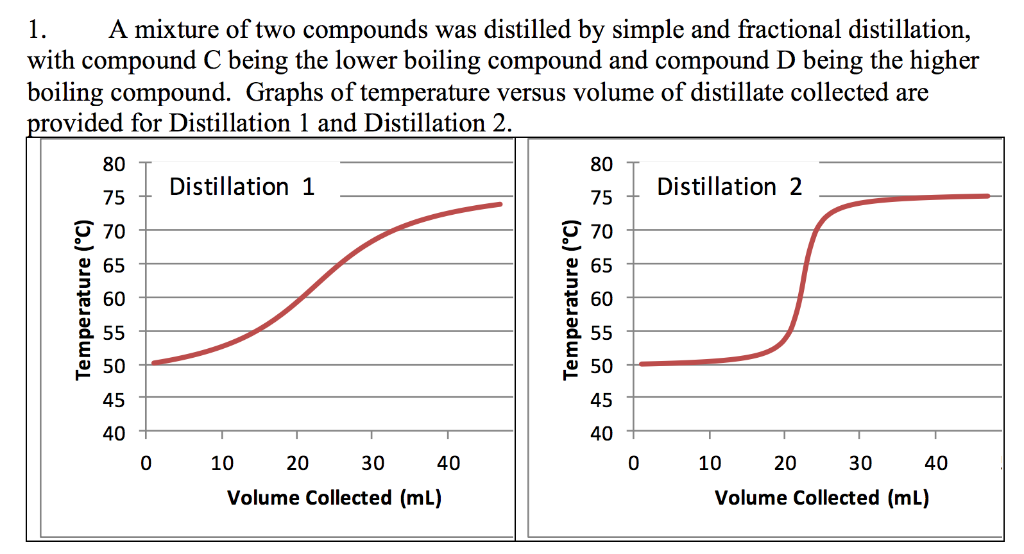(b)       Estimate the boiling points (b.p.) of compound C (lower boiling) and compound D (higher boiling)? Compound C b.p.                   °C      Compound D b.p.                     °C A mixture of two compounds was distilled by simple and fractional distillation, with compound C being the lower boiling compound and compound D being the higher boiling compound. Graphs of temperature versus volume of distillate collected are rovided for Distillation 1 and Distillation 2 80 75 O 70 2 65 60 2 55...

• ### 21. Which of the following compounds has the highest boiling poin A) A 22. Which of...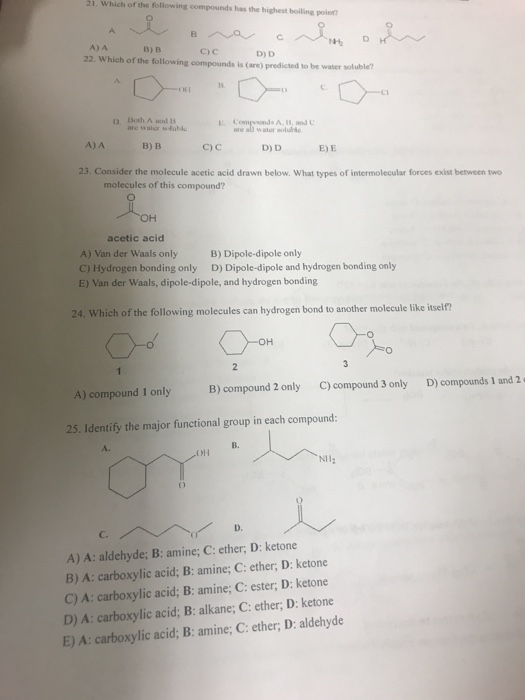21. Which of the following compounds has the highest boiling poin A) A 22. Which of the following compounds is (are) predicted to be water soluble? B) B с) с D) D Both A and 1 are walcr seluble ure all water soluhc A)A B)B C) C D) D E) E 23. Consider the molecule acetic acid drawn below. What types of intermolecular forces exist between two molecules of this compound? он acetic acid A) Van der Waals only C)...

• ### 2 Why is the boiling point of ammonia higher than the boiling point of ethane? What...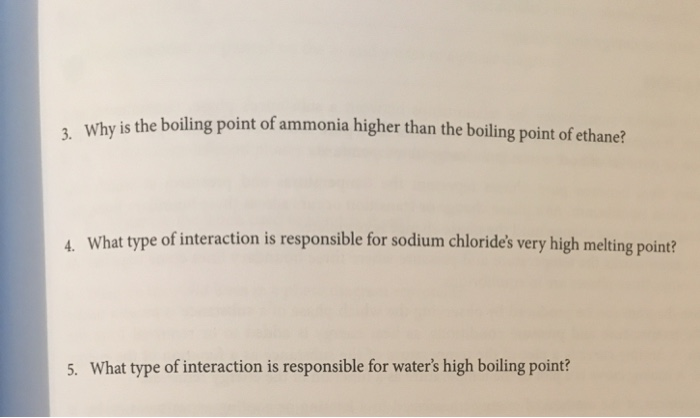2 Why is the boiling point of ammonia higher than the boiling point of ethane? What type of interaction is responsible for sodium chloride's very high melting point? A 5. What type of interaction is responsible for water's high boiling point? 4 A gaseous sample of a compound has a gas density of 0.647 g/L at 694 torr and 20 °C. What is the molar mass of this compound? If this compound contains only nitrogen and hydrogen, and is 82.2%...